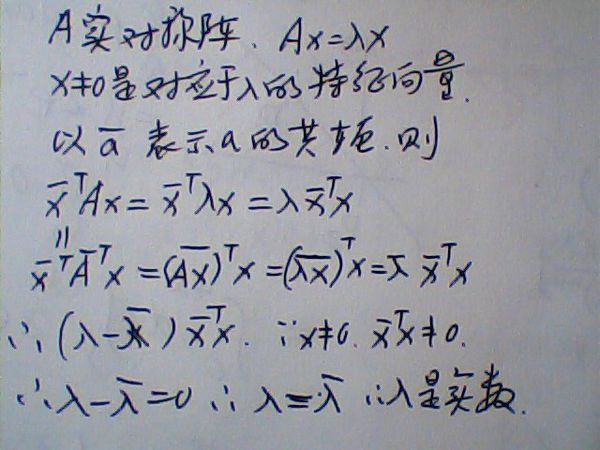## 谱聚类基础之二：相似矩阵

1. K邻近法是只要一个点在另一个点的K近邻中，则保留$s_{ij}$
1. K邻近法是必须两个点互为K近邻中，才能保留$s_{ij}$

## 谱聚类基础之三：拉普拉斯矩阵

1）拉普拉斯矩阵是对称矩阵，这可以由$D$和$W$都是对称矩阵而得。

2）由于拉普拉斯矩阵是对称矩阵，则它的所有的特征值都是实数。3）对于任意的向量$f$,我们有

4) 拉普拉斯矩阵是半正定的，且对应的n个实数特征值都大于等于0，即$0 =\lambda_1 \leq \lambda_2 \leq… \leq \lambda_n$， 且最小的特征值为0，这个由性质3很容易得出。

## 谱聚类基础之四：无向图切图## 谱聚类之切图聚类

### 6.1 RatioCut切图

RatioCut切图为了避免第五节的最小切图，对每个切图，不光考虑最小化$cut(A_1,A_2,…A_k)$，它还同时考虑最大化每个子图点的个数，即：

### 6.2 Ncut切图

Ncut切图和RatioCut切图很类似，但是把Ratiocut的分母$|Ai|$换成$vol(A_i)$.

$D^{-1/2}LD^{-1/2}$相当于对拉普拉斯矩阵$L$做了一次标准化，即$\frac{L_{ij}}{\sqrt{d_i*d_j}}$

## 谱聚类算法流程

1) 根据输入的相似矩阵的生成方式构建样本的相似矩阵S
2）根据相似矩阵S构建邻接矩阵W，构建度矩阵D
3）计算出拉普拉斯矩阵L
4）构建标准化后的拉普拉斯矩阵$D^{-1/2}LD^{-1/2}$
5）计算$D^{-1/2}LD^{-1/2}$最小的$k_1$个特征值所各自对应的特征向量$f$
6) 将各自对应的特征向量$f$组成的矩阵按行标准化，最终组成$n \times k_1$维的特征矩阵F
7）对F中的每一行作为一个$k_1$维的样本，共n个样本，用输入的聚类方法进行聚类，聚类维数为$k_2$。
8）得到簇划分$C(c_1,c_2,…c_{k_2})$.

## 谱聚类算法总结

1）谱聚类只需要数据之间的相似度矩阵，因此对于处理稀疏数据的聚类很有效。这点传统聚类算法比如K-Means很难做到
2）由于使用了降维，因此在处理高维数据聚类时的复杂度比传统聚类算法好。

1）如果最终聚类的维度非常高，则由于降维的幅度不够，谱聚类的运行速度和最后的聚类效果均不好。
2) 聚类效果依赖于相似矩阵，不同的相似矩阵得到的最终聚类效果可能很不同。

## 参考

------ 本文结束------